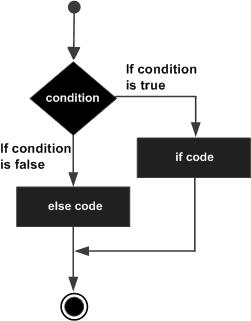if 语句由一个布尔表达式后跟一个或多个语句。if 条件为真，下面的 if 条件被执行的语句。if 条件为假，else部分的语句将被执行。

## 语法：

```If(boolean_expression) Then
Statement 1
.....
.....
Statement n
Else
Statement 1
.....
....
Statement n
End If```

## 流程图## 示例

```Private Sub if_demo_Click()
Dim x As Integer
Dim y As Integer

x = 234
y = 324

If x > y Then
MsgBox "X is Greater than Y"
Else
Msgbox "Y is Greater than X"
End If
End Sub```

`Y is Greater than X`